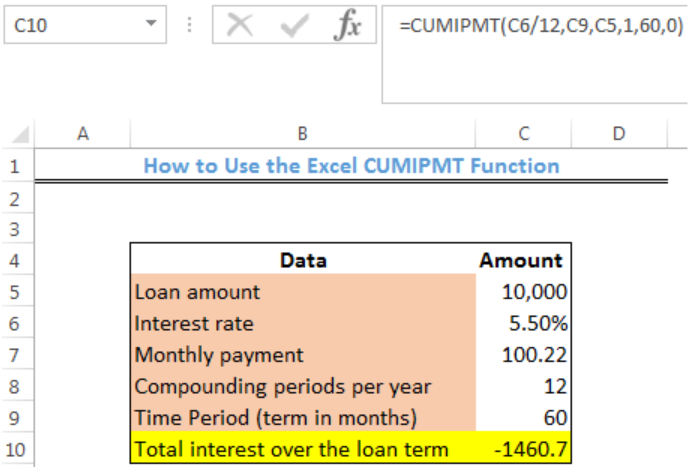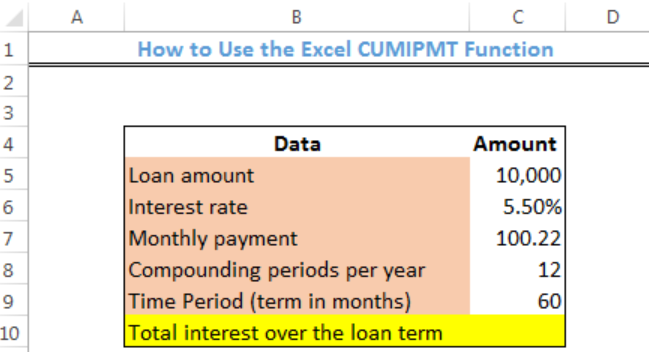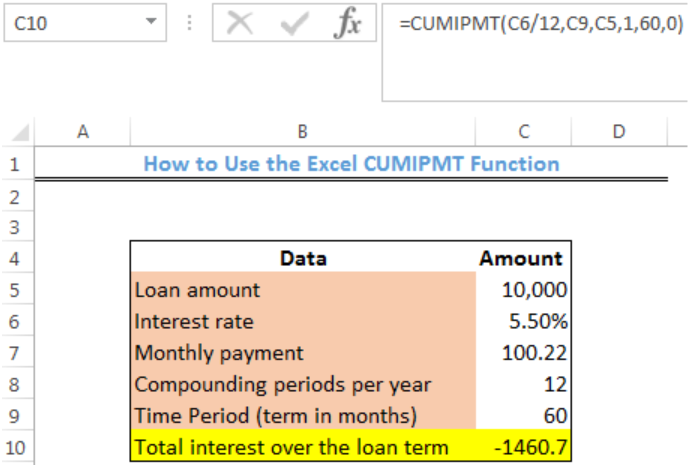Get instant live expert help with Excel or Google Sheets“My Excelchat expert helped me in less than 20 minutes, saving me what would have been 5 hours of work!”

#### Post your problem and you’ll get Expert help in seconds.

Your message must be at least 40 characters
Our professional Expert are available now. Your privacy is guaranteed.

# How to Use the Excel CUMIPMT Function

We can use the excel CUMIPMT function to return the cumulative interest to be paid on a loan from a start to an end period. This function is useful for calculating and verifying the total interest paid between any two payment periods. The steps below will walk through the process.Figure 1 – How to Use the Excel CUMIPMT Function

## Syntax

`=CUMIPMT(rate, nper, pv, start_period, end_period, type)`

• Rate– This is the interest rate per period (C6/12)
• Nper– This is the total number of payments for the loan. (C9)
• Pv– This is the present value or the total value of all payments at present (C5)
• Start_period– This represents the first payment in a calculation.
• End_period– This represents the last payment in a calculation.
• Type– When the payments are due. 0 = payment due at the end of a period. 1 = payment due at the beginning of a period.

## Formula

`=CUMIPMT(C6/12,C9,C5,1,60,0)`

## Setting up the Data

We will set up our table as shown in figure 2. We intend to calculate the cumulative interest paid on the loan amount for 60 months.

• We will place the parameters in the range: B5:B9.
• The figures will be contained in Column C
• The result will be returned in Cell C10Figure 2 – Setting up the Data

## Using the Excel CUMIPMT Function

• We will click on Cell C10
• We will insert the formula below into Cell C10
`=CUMIPMT(C6/12,C9,C5,1,60,0)`Figure 3 – Result of the Cumulative Interest Paid

## Instant Connection to an Expert through our Excelchat Service

Most of the time, the problem you will need to solve will be more complex than a simple application of a formula or function. If you want to save hours of research and frustration, try our live Excelchat service! Our Excel Experts are available 24/7 to answer any Excel question you may have. We guarantee a connection within 30 seconds and a customized solution within 20 minutes.

### Did this post not answer your question? Get a solution from connecting with the expert.Another blog reader asked this question today on Excelchat:## Subscribe to Excelchat.coAnother blog reader asked this question today on Excelchat: